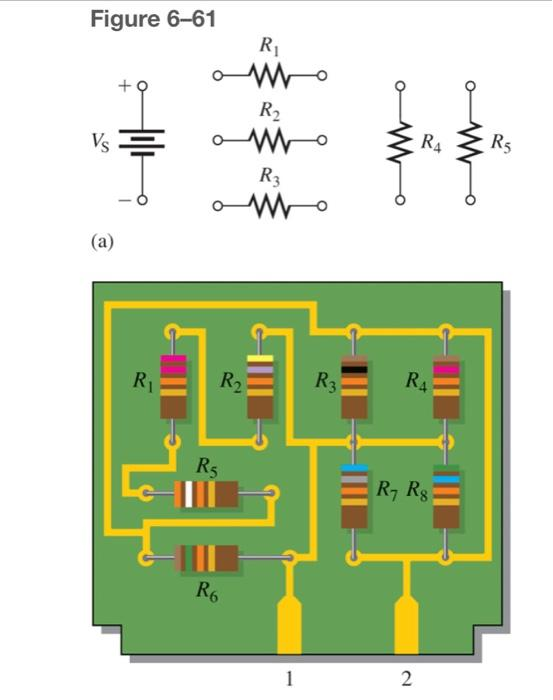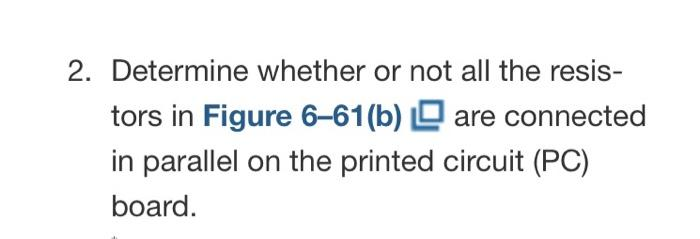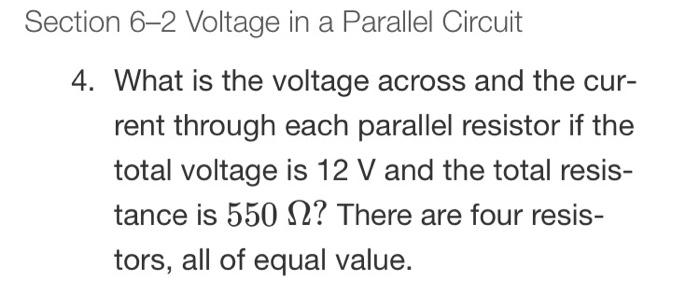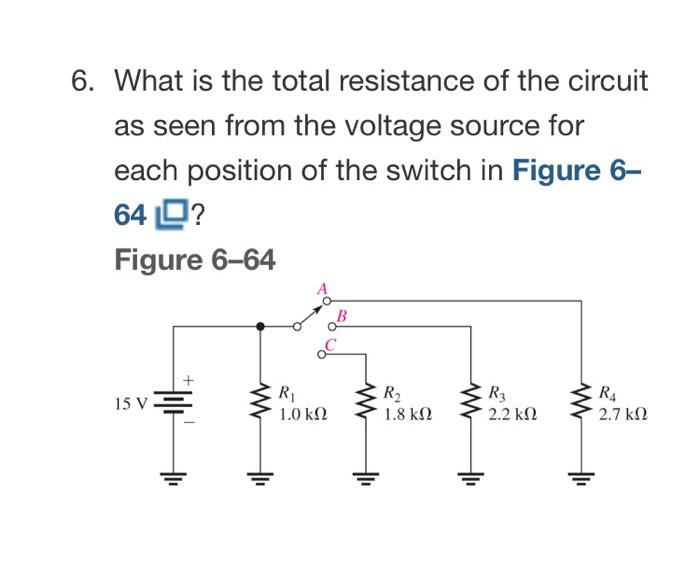# Question o Figure 6–61 R mo + R2 Vs amo R3 mo (a) W RA RS R R2 R3 R4 Rg R, Rg Ro 1 2 2. Determine whether or not all the resis- tors in Figure 6-61(b) Q are connected in parallel on the printed circuit (PC) board. Section 6–2 Voltage in a Parallel Circuit 4. What is the voltage across and the cur- rent through each parallel resistor if the total voltage is 12 V and the total resis- tance is 550 12? There are four resis- tors, all of equal value. 6. What is the total resistance of the circuit as seen from the voltage source for each position of the switch in Figure 6- 64 0? Figure 6-64 15 V R2 R 1.Ο ΚΩ ş R3 1.8 kΩ 2.2 k22 R4 2.7 k12WIKNOY The Asker · Electrical EngineeringTranscribed Image Text: o Figure 6–61 R mo + R2 Vs amo R3 mo (a) W RA RS R R2 R3 R4 Rg R, Rg Ro 1 2 2. Determine whether or not all the resis- tors in Figure 6-61(b) Q are connected in parallel on the printed circuit (PC) board. Section 6–2 Voltage in a Parallel Circuit 4. What is the voltage across and the cur- rent through each parallel resistor if the total voltage is 12 V and the total resis- tance is 550 12? There are four resis- tors, all of equal value. 6. What is the total resistance of the circuit as seen from the voltage source for each position of the switch in Figure 6- 64 0? Figure 6-64 15 V R2 R 1.Ο ΚΩ ş R3 1.8 kΩ 2.2 k22 R4 2.7 k12
More
Transcribed Image Text: o Figure 6–61 R mo + R2 Vs amo R3 mo (a) W RA RS R R2 R3 R4 Rg R, Rg Ro 1 2 2. Determine whether or not all the resis- tors in Figure 6-61(b) Q are connected in parallel on the printed circuit (PC) board. Section 6–2 Voltage in a Parallel Circuit 4. What is the voltage across and the cur- rent through each parallel resistor if the total voltage is 12 V and the total resis- tance is 550 12? There are four resis- tors, all of equal value. 6. What is the total resistance of the circuit as seen from the voltage source for each position of the switch in Figure 6- 64 0? Figure 6-64 15 V R2 R 1.Ο ΚΩ ş R3 1.8 kΩ 2.2 k22 R4 2.7 k12